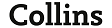# ninth definición, ninth significado | diccionario de inglés definición

Buscar también en: Web Noticias Enciclopedia Imágenes## ninth

1    usually prenominal
a    coming after the eighth in counting order, position, time, etc.; being the ordinal number of nine. Often written: 9th
b    (as n)
he came on the ninth, ninth in line
n
2
a    one of nine equal or nearly equal parts of an object, quantity, measurement, etc.
b    (as modifier)
a ninth part
3    the fraction equal to one divided by nine (1/9)
4      (Music)
a    an interval of one octave plus a second
b    one of two notes constituting such an interval
c       See       ninth chord
5      (Also)    ninthly   after the eighth person, position, event, etc.
sentence connector
6      (Also)    ninthly   as the ninth point: linking what follows to the previous statement
(Old English nigotha; related to Old High German niunto, Old Norse niundi)

ninth chord
n   a chord much used in jazz and pop, consisting of a major or minor triad with the seventh and ninth added above the root,   (Often shortened to)    ninthninth chord
n   a chord much used in jazz and pop, consisting of a major or minor triad with the seventh and ninth added above the root,   (Often shortened to)    ninth

ninth
1    usually prenominal
a    coming after the eighth in counting order, position, time, etc.; being the ordinal number of nine. Often written: 9th
b    (as n)
he came on the ninth, ninth in line
n
2
a    one of nine equal or nearly equal parts of an object, quantity, measurement, etc.
b    (as modifier)
a ninth part
3    the fraction equal to one divided by nine (1/9)
4      (Music)
a    an interval of one octave plus a second
b    one of two notes constituting such an interval
c       See       ninth chord